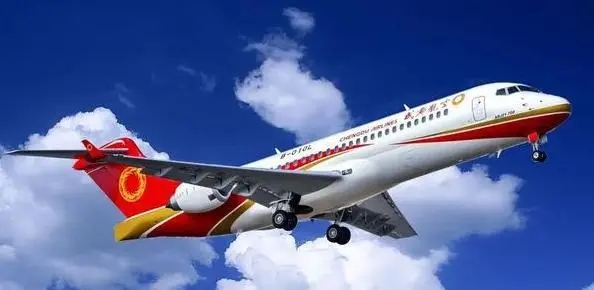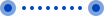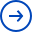# 重泡比怎么计算价格（航空运费如何计算）

2022年12月13日 16:29:33 更新 2329 浏览 作者：国际快递-百运网计算航空运费时，我们常常听到“计费重量”的说法：计费重量Chargeable Weight = 毛重(Gross Weight)和体积重量(Volume Weight)二者取重者。体积重量 = 长cm X宽cm X高cm / 6000KG/cm³计费重量 = 毛重时，为重货;反之，计费重量 = 体积重量时，为泡货。

此时：

泡重比=6000cm³/KG=0.006m³/0.001t=6m³/t

重泡比=1kg/6000cm³=1kg/0.006m³=167kg/m³

(一)平货计费重量测算

标准平货1:167,实际重量/体积重=1

收费重量=实际重量=体积重=167公斤/立方

收费标准：可以以此价格的吨上价格为参照收费标准。

(二)泡货计费重量测算公式

货代给客户X/Y分泡，X+Y=10,(其中X、Y为整数)。

应分重量：体积重(体积*167)-实际重

客户承担：(体积重-实际重)*X/(X+Y)

货代承担：(体积重-实际重)*Y/(X+Y)

货代收取运费标准：

体积重-(体积重-实际重)Y/(X+Y) =X/(X+Y)

体积重+Y/(X+Y)实际重

(三)重货计费重量测算

1、重货1:200,实际重/体积重=1.2

收费重量=实际重量=1.2体积重

2、货1:300,实际重/体积重=1.8收费重量=实际重量=1.8体积重

(四)重泡货物配载

1.若泡货1:60，实际重/体积重=0.36 四六分泡

收费重量=X/(X+Y)体积重+Y/(X+Y)实际重

=X/(X+Y)体积重+Y/(X+Y)*体积重*0.6

=体积重*(4+0.36*6)/10

=0.616体积重

=102.67公斤/立方

重货配载：

同样体积的1:232重货配载;

一半体积的1:300重货配载。

2.若泡货1:100，实际重/体积重=0.6

收费重量=X/(X+Y)体积重+Y/(X+Y)实际重

=X/(X+Y)体积重+Y/(X+Y)*体积重*0.6

=体积重*(X+0.6Y)/(X+Y)

三七分泡收费重量=体积重*(3+0.6*7)/10

=0.72体积重

=0.72*166.67

=120.00公斤/立方

重货配载：

同样体积的1:215重货配载;

一半体积的1:260重货配载。

3.泡货1:150，实际重/体积重=0.9

收费重量=X/(X+Y)体积重+Y/(X+Y)实际重

=X/(X+Y)体积重+Y/(X+Y)*体积重*0.9

=体积重*(X+0.9Y)/(X+Y)

二八分泡

收费重量=体积重量*(2+0.9*8)/10

=0.92体积重

=0.92*166.67

=153.34公斤/立方

重货配载：同样体积的1:180重货配载;

一半体积的1:200重货配载。

• 1
• 2
• 3
• 4
• 5
• 6
• 7
• 8
• 9
• 10FBA

ShenzhenUnited Kingdom

¥48.40ShenzhenItaly

¥52.78Shenzhen¥52.44ShenzhenAustralia

¥23.20ShenzhenGermany

¥52.78